#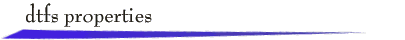A real, N-periodic, discrete-time signal x[n] can be represented as a discrete-time Fourier series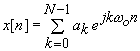where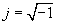, and the discrete-time fundamental frequency is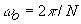.

The complex coefficients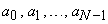can be calculated from the expression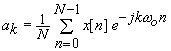The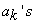are called the spectral coefficients of the signal x[n].  A plot of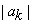vs k is called the magnitude spectrum of x[n], and a plot of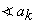vs k is called the phase spectrum of x[n]. These plots, particularly the magnitude spectrum, provide a picture of the frequency composition of the signal. Recall that the spectral coefficients for a periodic signal repeat according to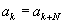.

The applet below illustrates properties of the discrete-time Fourier series. You can enter a value of  N up to 15 and specify x[n] by sketching the signal or by sketching magnitude and phase spectra with the mouse. Then standard operations can be performed on x[n] and the effects on the signal and its spectra are displayed. (Click the Reset button between operations.)

Let y[n]  be the signal resulting from an operation on x[n], and let the discrete-time Fourier series coefficients of y[n] be specified by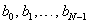. The table below lists the operations available, where M and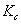are integers and c is a real constant. For the ideal low pass filter,specifies the highest frequency,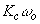, that is passed by the filter. For the ideal low pass filter,specifies the lowest frequency passed by the filter.

## Operation

Resulting signal y[n]

Spectral coefficients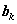of y[n]

k=0, 1,…, N-1

Amplitude Scale

c x[n]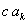Time Shift

x[n – M]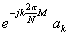Time Scale*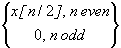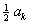Time Reverse

x[- n]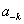First Difference

x[n] -  x[n-1]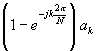Running Sum**

x[n]+ x[n-1]+ x[n-2]+¼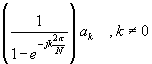Ideal Low Pass

filtered version of x[n],

cutoff frequency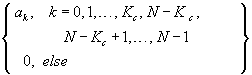Ideal High Pass

filtered version of x[n],

cutoff frequency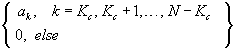*    For this time scaling, the period of y[n] is 2N.

**  For the running sum, y[n] is periodic if and only if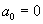.return to demonstrations page

 Applet by Lan Ma.